## COMMON I.C.'S PIN ASSIGNMENTS AND LOGIC

### 7432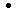74S3274LS3274F32 QUAD 2-INPUT OR GATE

PIN ASSIGNMENT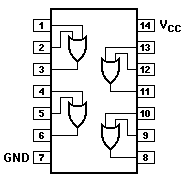LOGIC DIAGRAM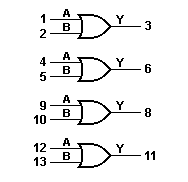TRUTH TABLE

INPUTS OUTPUT
A B Y
L
L
H
H
L
H
L
H
L
H
H
H

H = HIGH voltage level
L = LOW voltage level

### 747474S7474LS7474F74 DUAL D-TYPE FLIP-FLOP (POSITIVE EDGE TRIGGERED)

PIN ASSIGNMENT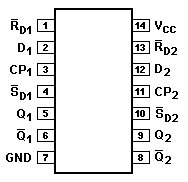LOGIC DIAGRAM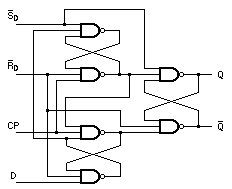TRUTH TABLE

OPERATING MODE INPUTS OUTPUTSSDRD
CP D QQ
Asyn. Set
Asyn. Reset (Clear)
Undetermined (a)
L
H
L
H
H
H
L
L
H
H
X
X
X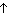X
X
X
h
l
H
L
H
H
L
L
H
L
L
H

H = HIGH voltage level steady state.
h = HIGH voltage level one setup time prior to the LOW-to-HIGH clock transition.
L = LOW voltage level steady state.
l = LOW voltage level one setup time prior to the LOW-to-HIGH clock transition.
X = don't care.= LOW-to-HIGH clock transition.

NOTE
 (a) Both outputs will be HIGH while bothSD andRD are LOW. But the output states are unpredictable ifSD andRD go HIGH simultaneously.

### 7412374LS123 DUAL RETRIGGERABLE MONOSTABLE MULTIVIBRATOR

PIN ASSIGNMENT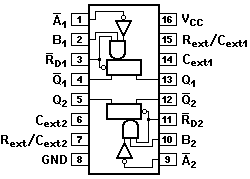LOGIC DIAGRAM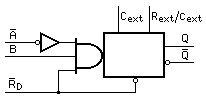TRUTH TABLE

INPUTS OUTPUTSRDA
B QQ
L
X
X
H
HX
H
X
L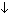L
X
X
LH
H
L
L
L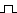H
H
H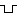H = HIGH voltage level
L = LOW voltage level
X = Don't care= LOW-to-HIGH transition= HIGH-to-LOW transition= One HIGH-level pulse= One LOW-level pulse